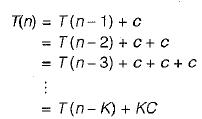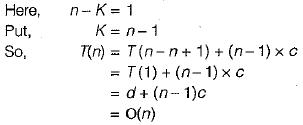Courses

# Algorithm Analysis And Asymptotic Notation (Advance Level) - 1

## 15 Questions MCQ Test Question Bank for GATE Computer Science Engineering | Algorithm Analysis And Asymptotic Notation (Advance Level) - 1

Description
This mock test of Algorithm Analysis And Asymptotic Notation (Advance Level) - 1 for Computer Science Engineering (CSE) helps you for every Computer Science Engineering (CSE) entrance exam. This contains 15 Multiple Choice Questions for Computer Science Engineering (CSE) Algorithm Analysis And Asymptotic Notation (Advance Level) - 1 (mcq) to study with solutions a complete question bank. The solved questions answers in this Algorithm Analysis And Asymptotic Notation (Advance Level) - 1 quiz give you a good mix of easy questions and tough questions. Computer Science Engineering (CSE) students definitely take this Algorithm Analysis And Asymptotic Notation (Advance Level) - 1 exercise for a better result in the exam. You can find other Algorithm Analysis And Asymptotic Notation (Advance Level) - 1 extra questions, long questions & short questions for Computer Science Engineering (CSE) on EduRev as well by searching above.
QUESTION: 1

### f{n) = 0 (g (n)) if and only if

Solution: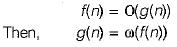QUESTION: 2

### Look at the following algorithms int n; int A; void (x){ int i; for (i=n/2;i > = 1; i -- ) y(i); } } void  y(int i) { .........; .........; .........; } Let complexity of y is O(log2n). Then the complexity of x will be

Solution:

In this programme for loop run n/2 times and in this ‘for loop’ y(i) have (log2 n) time complexity. In the above we will select maximum time complexity i.e., (n/2) log2n.
Then the total time complexity for the ‘for loop’ will O(n logn).

QUESTION: 3

### The time complexity of the following C function is (assume n > 0) int recursive (int n) { if (n == 1) return(1); else return(recursive(n- 1) + recursive(n - 1)); }

Solution:

The given C function is recursive. The best way to find the time complexity of recursive function is that convert the code (algorithm) into recursion equation and solution of the recursion equation is the time complexity of given algorithm.

1. int recursive (int i){
2 . if ( n == 1) return (1);
3. else
4. return (recursive(n-1) + recursive(n-1));
5. }
Let recursive(n) - T(n)
According to line 2 if (n = = 1) return(1) then the recursive equation is
T(n) = 1, n = 1
According to line 4 the recursion equation is
T(n) = T ( n - 1 ) + T(n-1), n > 1
So the complete recursion equation is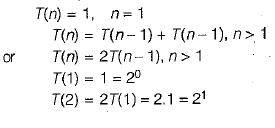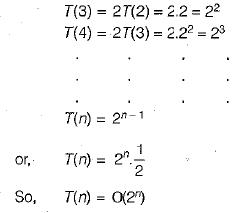QUESTION: 4

The recurrence equation: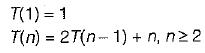Evaluates to

Solution: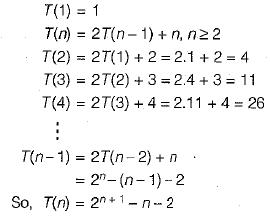QUESTION: 5

Let T(n) be a function defined by the recurrence

T(n) - 2T(n/2) + √n for n≥2 and T(1)  = 1

Which of the following statements is TRUE?

Solution: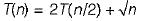By substitution method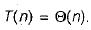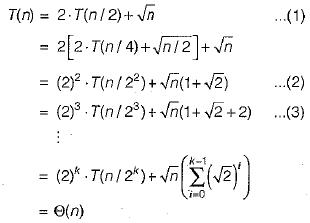QUESTION: 6

Suppose T(n) = 2T(n/2) + n, T(0) = T(1) = 1 Which one of the following is FALSE?

Solution:

T(0)= T(1) = 1
T(n) = 2T(n/2) + n
T(n) be computed as follows: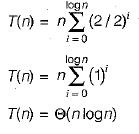[T(n) can also proved by Master Theorem]
if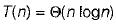then it is also 0(n logn) and O(n2) but it is not Ω(n2).

QUESTION: 7

Consider the following segment of C code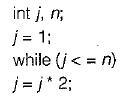The number of comparisons made in the execution of the loop for any n > 0 is

Solution:

Let the increment of j is 2°, 21...., 2i for some value of i so according to the question for while loop: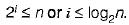One extra comparison required for the termination of while loop. So total number of comparisons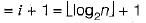QUESTION: 8

What is the time complexity of the following recursive function:
int DoSomething ( int n)
{
if {n< = 2)
return 1;
else
return DoSomething (floor (sqrt (n)))+n;
}

Solution:

The given function is recursive so the equivalent recursion equation is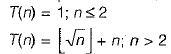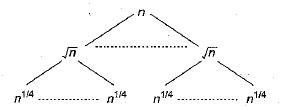All the level sums are equal to n. The problem size at level k of the recursion tree is n2-k and we stop recursing when this value is a constant. Setting n2-k = 2 and solving for k gives us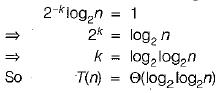QUESTION: 9

Using the standard algorithm, what is the time required to determine that a number n is prime?

Solution:

It takes not more than n/2 comparison to check whether the given number n is prime or not.

QUESTION: 10

The running time of an algorithm T(n), where ‘n’ is the input size is given by

T(n) = 8T(n/2) + qn. if n> 1
p, if n = 1

where p, q are constants. The order of this algorithm is

Solution: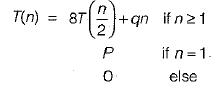Since q is constant, so our recurrence relation look like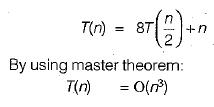QUESTION: 11

Let m, n be positive integers. Define Q(m, n) as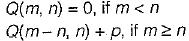Then Q(m, 3) is (a div b, gives the quotient when a is divided by b)

Solution:

Two cases: Q(m, 3)
(i) m ≥ n : here m ≥ 3
For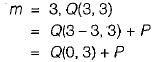∵ Since 3 is divide by 3 only 1 time = 0 + P= P.
So this recursive program generate P, m/n times until m < n.
So, Q(m, 3) = Px(m div 3)

QUESTION: 12

T(n) = T(2n/3) + 1 then T(n) is equal to

Solution: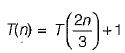By using recursive tree method: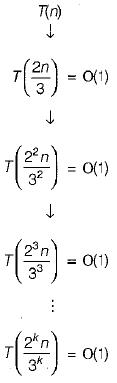So, height of tree is log(3/2)n
So, T(n) = Height x Work at each level.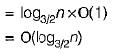QUESTION: 13

An algorithm is made up of 2 m odules m1 and m2. If order of M1 is f(n) and M2 is g(n) then the order of the algorithm is

Solution: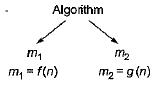So, order of algorithm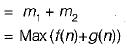QUESTION: 14

The recurrence relation T(n) = mT(n/2) + an2 is satisfied by

Solution:

T(n) = mT (n/2) + an2
Since,
m ≥ 5
So by applying Master Theorem: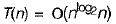QUESTION: 15

The running time T(n) where ‘n' is the input size of a recursive algorithm is given as follow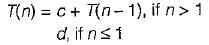The order of this algorithm is

Solution: